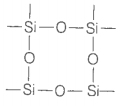Explain the following

1.  CO2 is a gas whereas SiO2 is a solid.

2.  Silicon forms ${\mathrm{SiF}}_{6}^{2-}$ ion whereas corresponding fluoro compound of carbon is not known.

(a) CO2 has a linear structure. Its dipole moment is zero. It is believed that CO2 molecule is a resonance hybrid has the following structures.
$\mathrm{O}=\mathrm{C}=\mathrm{O}↔{\mathrm{O}}^{-}-\mathrm{C}\equiv {\mathrm{O}}^{+}↔{\mathrm{O}}^{+}\equiv \mathrm{C}-{\mathrm{O}}^{-}$
The CO2 molecules are held together by weak van der Waals' forces and thus, it exists as gas. In SiO2, due to large electronegative difference between Si and O, the Si-O bonds have considerable ionic nature.
Therefore, silica has three dimensional network like structure in which Si-atom is tetrahedrally bonded to four oxygen atoms and each oxygen atom is bonded to two silicon atoms by covalent bonds.There is not discrete SiO2 molecule. It is a network solid with octahedral coordination.
(b) In silicon, vacant 3d orbitals are available due to which it can accommodate electrons from 6 fluorine atoms, thereby forming ${\mathrm{SiF}}_{6}^{2-}$ ion. However, in case of C only 2p2 filled orbitals are available thus, it cannot expand their covalency more than 4. Thus, ${\mathrm{CCl}}_{6}^{2}$ is not known.